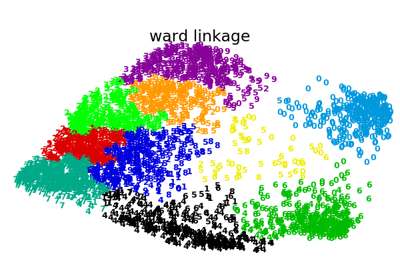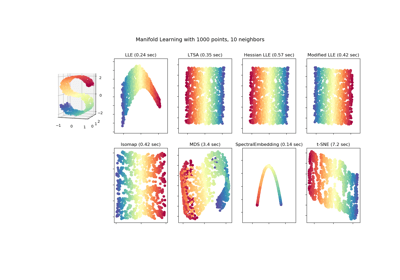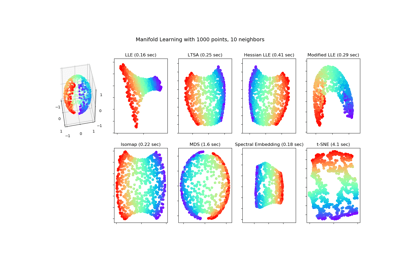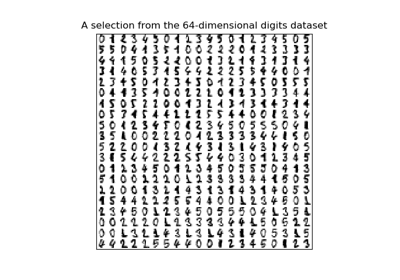/scikit-learn

# sklearn.manifold.SpectralEmbedding

`class sklearn.manifold.SpectralEmbedding(n_components=2, affinity=’nearest_neighbors’, gamma=None, random_state=None, eigen_solver=None, n_neighbors=None, n_jobs=None)` [source]

Spectral embedding for non-linear dimensionality reduction.

Forms an affinity matrix given by the specified function and applies spectral decomposition to the corresponding graph laplacian. The resulting transformation is given by the value of the eigenvectors for each data point.

Note : Laplacian Eigenmaps is the actual algorithm implemented here.

Read more in the User Guide.

Parameters: `n_components : integer, default: 2` The dimension of the projected subspace. `affinity : string or callable, default` How to construct the affinity matrix. ‘nearest_neighbors’ : construct affinity matrix by knn graph ‘rbf’ : construct affinity matrix by rbf kernel ‘precomputed’ : interpret X as precomputed affinity matrix callable : use passed in function as affinity the function takes in data matrix (n_samples, n_features) and return affinity matrix (n_samples, n_samples). `gamma : float, optional, default` Kernel coefficient for rbf kernel. `random_state : int, RandomState instance or None, optional, default: None` A pseudo random number generator used for the initialization of the lobpcg eigenvectors. If int, random_state is the seed used by the random number generator; If RandomState instance, random_state is the random number generator; If None, the random number generator is the RandomState instance used by `np.random`. Used when `solver` == ‘amg’. `eigen_solver : {None, ‘arpack’, ‘lobpcg’, or ‘amg’}` The eigenvalue decomposition strategy to use. AMG requires pyamg to be installed. It can be faster on very large, sparse problems, but may also lead to instabilities. `n_neighbors : int, default` Number of nearest neighbors for nearest_neighbors graph building. `n_jobs : int or None, optional (default=None)` The number of parallel jobs to run. `None` means 1 unless in a `joblib.parallel_backend` context. `-1` means using all processors. See Glossary for more details. `embedding_ : array, shape = (n_samples, n_components)` Spectral embedding of the training matrix. `affinity_matrix_ : array, shape = (n_samples, n_samples)` Affinity_matrix constructed from samples or precomputed.

#### Examples

```>>> from sklearn.datasets import load_digits
>>> from sklearn.manifold import SpectralEmbedding
>>> X.shape
(1797, 64)
>>> embedding = SpectralEmbedding(n_components=2)
>>> X_transformed = embedding.fit_transform(X[:100])
>>> X_transformed.shape
(100, 2)
```

#### Methods

 `fit`(X[, y]) Fit the model from data in X. `fit_transform`(X[, y]) Fit the model from data in X and transform X. `get_params`([deep]) Get parameters for this estimator. `set_params`(**params) Set the parameters of this estimator.
`__init__(n_components=2, affinity=’nearest_neighbors’, gamma=None, random_state=None, eigen_solver=None, n_neighbors=None, n_jobs=None)` [source]
`fit(X, y=None)` [source]

Fit the model from data in X.

Parameters: `X : array-like, shape (n_samples, n_features)` Training vector, where n_samples is the number of samples and n_features is the number of features. If affinity is “precomputed” X : array-like, shape (n_samples, n_samples), Interpret X as precomputed adjacency graph computed from samples. `self : object` Returns the instance itself.
`fit_transform(X, y=None)` [source]

Fit the model from data in X and transform X.

Parameters: `X : array-like, shape (n_samples, n_features)` Training vector, where n_samples is the number of samples and n_features is the number of features. If affinity is “precomputed” X : array-like, shape (n_samples, n_samples), Interpret X as precomputed adjacency graph computed from samples. `X_new : array-like, shape (n_samples, n_components)`
`get_params(deep=True)` [source]

Get parameters for this estimator.

Parameters: `deep : boolean, optional` If True, will return the parameters for this estimator and contained subobjects that are estimators. `params : mapping of string to any` Parameter names mapped to their values.
`set_params(**params)` [source]

Set the parameters of this estimator.

The method works on simple estimators as well as on nested objects (such as pipelines). The latter have parameters of the form `<component>__<parameter>` so that it’s possible to update each component of a nested object.

Returns: self

## Examples using `sklearn.manifold.SpectralEmbedding`Various Agglomerative Clustering on a 2D embedding of digitsComparison of Manifold Learning methodsManifold Learning methods on a severed sphereManifold learning on handwritten digits: Locally Linear Embedding, Isomap…